# Exercise 15.2 Visualizing Solid Shapes NCERT Solutions Class 7

## Chapter 15 Ex.15.2 Question 1

Use isometric dot paper and make an isometric sketch for each one of the given shapes: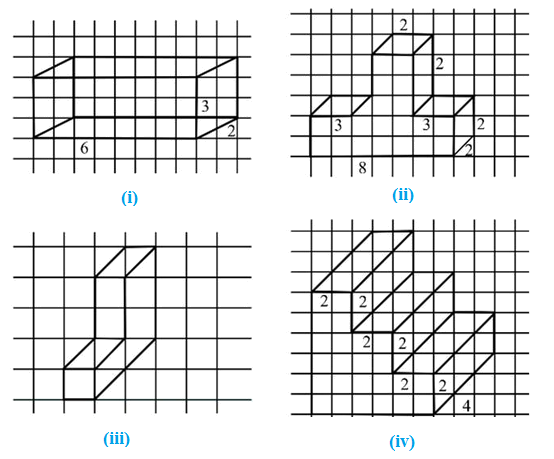### Solution

What is known?

Oblique sketch

What is unknown?

Isometric sketch

Reasoning:

See the figure carefully and then draw it on isometric dot paper.

Steps: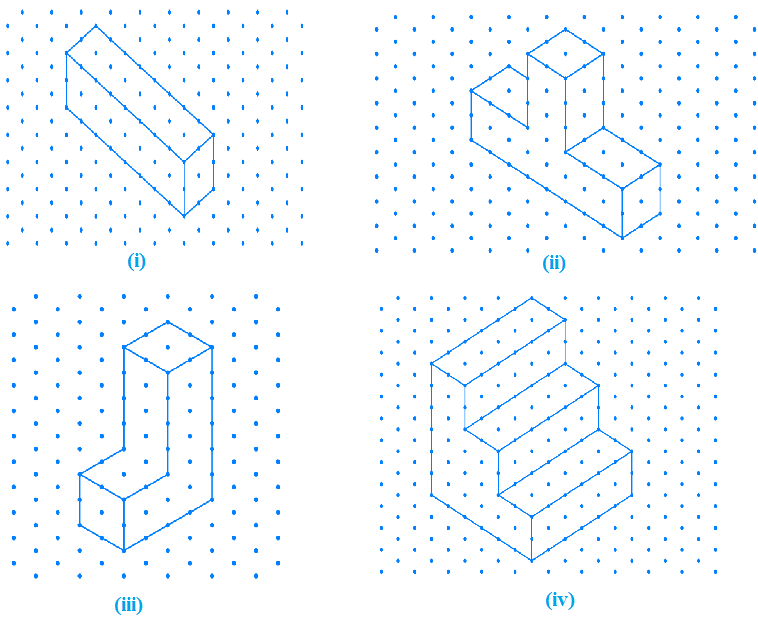## Chapter 15 Ex.15.2 Question 2

The dimensions of a cuboid are $$5$$ cm, $$3$$ cm and $$2$$ cm. Draw three different isometric sketches of this cuboid.

### Solution

What is known?

Dimensions of a cuboid.

What is unknown?

$$3$$ different isometric sketches of this cuboid.

Reasoning:

Draw $$3$$ rough sketch of cuboid having dimensions of $$5\,\rm{cm}, 2\,\rm{cm}$$ and $$3\, \rm{cm}$$ then draw it on isometric dot paper.

Steps:

The dimensions of cuboid are $$5$$ cm, $$3$$ cm and $$2$$ cm: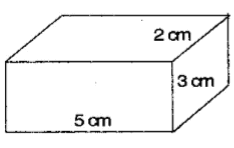Three different isometric sketches of given cuboid are below: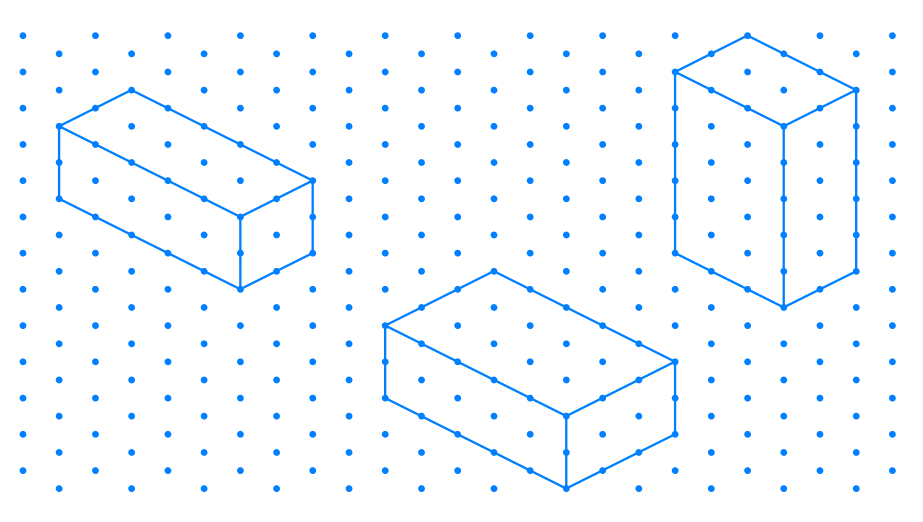## Chapter 15 Ex.15.2 Question 3

Three cubes each with $$2$$ cm edge are placed side by side to form a cuboid. Sketch an oblique or isometric sketch of this cuboid.

### Solution

What is known?

Three cubes each with $$2 \,\rm{cm}$$ edge are placed side by side to form a cuboid.

What is unknown?

Oblique or isometric sketch of this cuboid.

Reasoning:

Draw rough sketch of this cuboid and then draw oblique and isometric sketch.

Steps:

Length of three each cube edge $$=$$ $$2$$ cm

Placing three cubes side by side, then the dimension of cuboid $$= 6\rm{cm}×2 \rm{cm}×2 \rm{cm}$$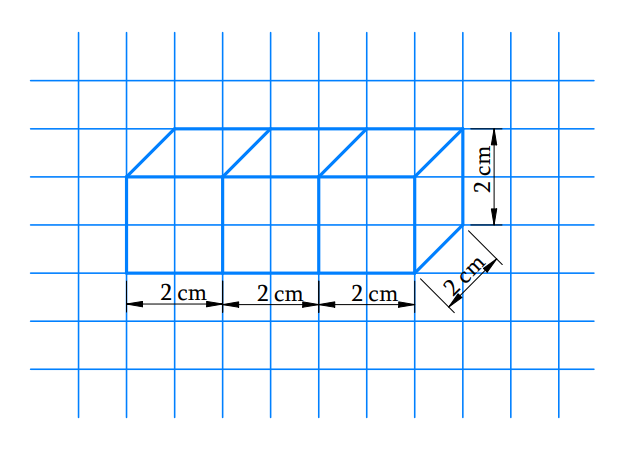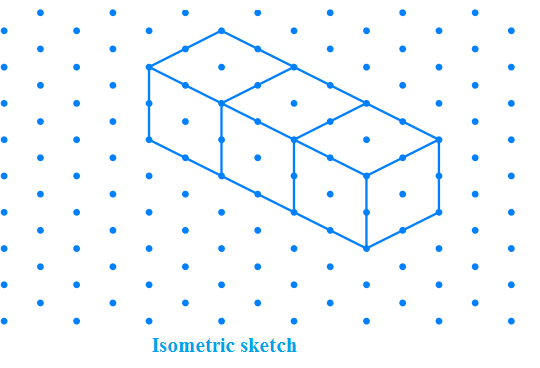## Chapter 15 Ex.15.2 Question 4

Make an oblique sketch for each one of the given isometric shapes: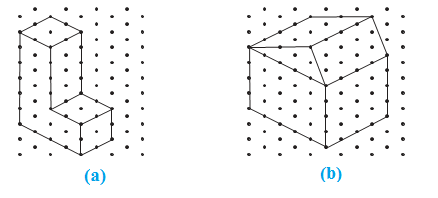### Solution

What is known?

isometric sketch

What is unknown?

Oblique sketch

Reasoning:

See the figure carefully and then draw an oblique sketch of these figures.

Steps:

Below are the oblique sketches of given isometric shapes: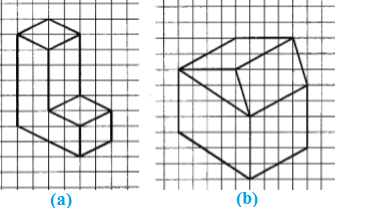## Chapter 15 Ex.15.2 Question 5

Give (i) an oblique sketch and (ii) an isometric sketch for each of the following:

(a) A cuboid of dimensions $$5$$ cm, $$3$$ cm and $$2$$ cm. (Is your sketch unique?)

(b) A cube with an edge $$4$$ cm long.

An isometric sheet is attached at the end of the book. You could try to make on it some cubes or cuboids of dimensions specified by your friend.

### Solution

What is known?

Dimensions of the cube and cuboid.

What is unknown?

Oblique and isometric sketch of cube and cuboid.

Reasoning:

First draw a rough sketch then draw oblique and isometric sketch for cube and cuboid.

Steps:

a) oblique and isometric sketch for a cuboid of dimensions $$5$$ cm, $$3$$ cm and $$2$$ cm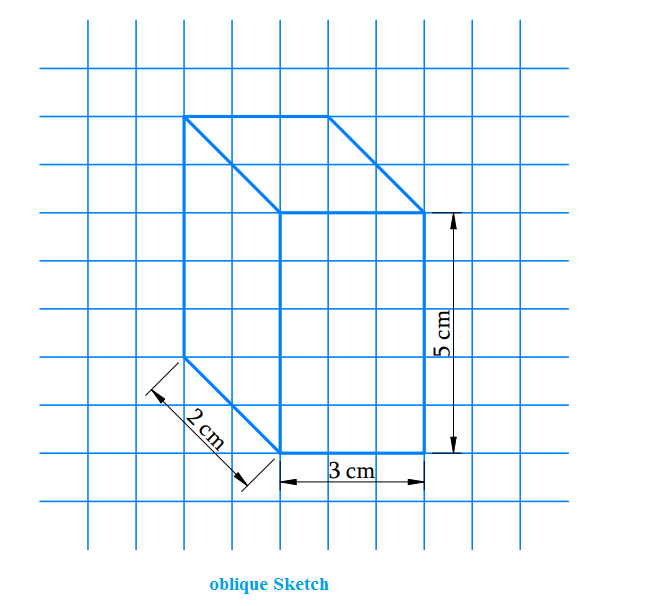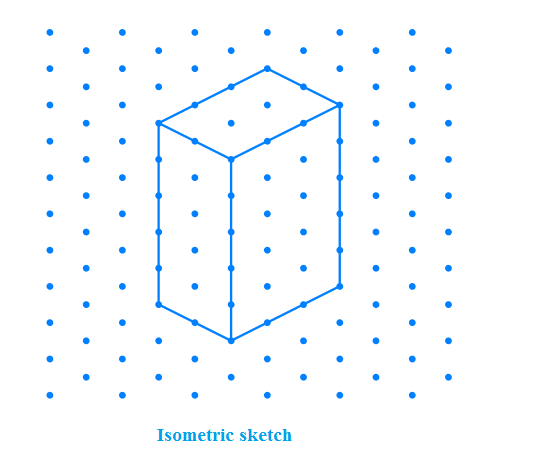b) oblique and isometric sketch for a cube with  $$4$$ cm length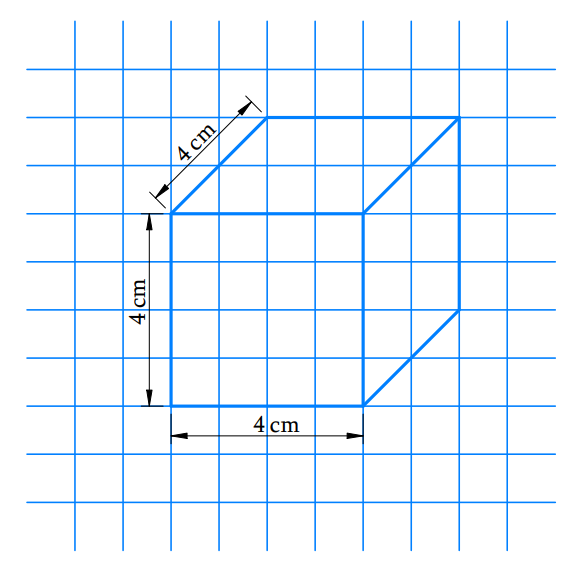Instant doubt clearing with Cuemath Advanced Math Program